Next: Pressure, Incompressibility and Asymmetry Up: Infinite Nuclear Matter Previous: Fermi surfaces and kinetic

## Equation of state'' of asymmetric polarized nuclear matter

In INM
. We choose pure neutron and proton states, which leads to
,
, and similarly for all other densities. We take the z axis as the quantization axis for the spin, i.e., st, x = st, y = 0, st := st, z, and for the kinetic spin density
. As discussed in Refs. , this breaks the isotropy of INM, leading to an axially deformed Fermi surface, an effect which we neglect. Adding the kinetic term, the total energy per nucleon (i.e. the equation of state'') for the energy functional (7) and (8) is given by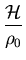=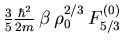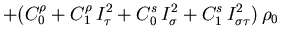(45)

For unpolarized INM one has
which recovers the expression given in Ref..

An interesting special case is polarized neutron matter, which is discussed in  for the Skyrme interactions. A stability criterion derived there from the two-body force point of view as outlined in Appendix 8 was used to constrain the parameters of the SLyx forces [23,24]. In this limiting case, one has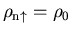,
, which is equivalent to
and leads to=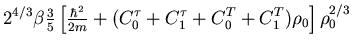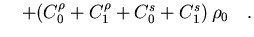(46)

Expressions (34) for an antisymmetrized Skyrme force imply that
, and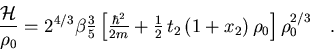(47)

The stability of polarized neutron matter for all densities requires
, so the SLyx interactions take
[23,24]. However, from the energy-density-functional point of view, the coupling constants are independent, and the second term in Eq. (50) also contributes to the stability condition.Next: Pressure, Incompressibility and Asymmetry Up: Infinite Nuclear Matter Previous: Fermi surfaces and kinetic
Jacek Dobaczewski
2002-03-15